﻿ 基于CFD对气体静压径向轴承动态分析 Dynamic Analysis of Radial Throttle Pinhole Hydrostatic Bearing Based on CFD

International Journal of Fluid Dynamics
Vol. 06  No. 04 ( 2018 ), Article ID: 28079 , 8 pages
10.12677/IJFD.2018.64019

Dynamic Analysis of Radial Throttle Pinhole Hydrostatic Bearing Based on CFD

Chou Fan, Shanghan Gao, Bing Li, Zhuang Ni

School of Mechanical Engineering, Guangxi University of Science and Technology, Liuzhou Guangxi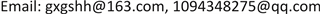Received: Nov. 23rd, 2018; accepted: Dec. 11th, 2018; published: Dec. 18th, 2018ABSTRACT

In view of the limitations of static analysis of static pressure bearings with radial throttling holes, a large eddy simulation (LES) is proposed to analyze the flow field changes near the aperture of a small hole between adjacent moments. At the same time, the changes in the flow field near the throttle holes in the circumferential position are analyzed, and the small hole throttle Kong Fu is obtained. The relationship between the parameters and the velocity of the eyes, the number of Maher and the variation of the vorticity of the eyes, is obtained by the analysis of the different parameters at different positions. Finally, we get the conclusion that the fluctuation of each parameter is related to the vibration of aerostatic bearing.

Keywords:Aerostatic Bearing, Large Eddy Simulation, Flow Field, Eddy Current, Vortex Line, Maher Number1. 引言

2. 基本方程

$\frac{\partial }{\partial t}+\frac{\partial p}{\partial {x}_{i}}\left(\rho {u}_{i}\right)=0$ (1)

$\frac{\partial }{\partial t}\left(\rho {u}_{i}\right)+\frac{\partial p}{\partial {x}_{j}}\left(\rho {u}_{i}{u}_{j}\right)=\frac{\partial {\tau }_{ij}}{\partial {x}_{j}}-\frac{\partial p}{\partial {x}_{i}}-\frac{\partial }{\partial {x}_{j}}\left({\delta }_{ij}\right)$ (3)

$\frac{\partial p}{\partial {x}_{i}}\left(\rho h{u}_{i}\right)=\frac{\partial \left(\rho {u}_{i}\right)}{\partial {x}_{i}}+{\tau }_{ij}\frac{\partial {u}_{i}}{\partial {x}_{i}}$ (3)

(3)是能量守恒方程

3. CFD仿真

3.1. 建立模型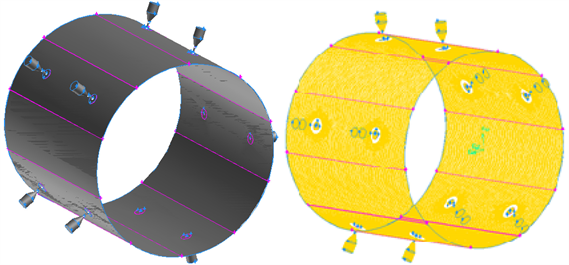Figure 1. The model of gas basin and the position of throttle hole

3.2. 网格划分

3.3. 边界条件及初始化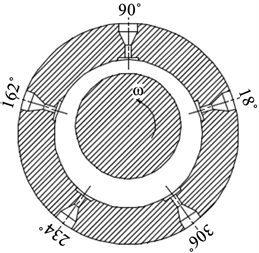(a) 定义节流孔附近条件和监测点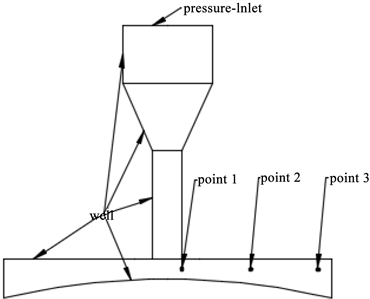(b) 节流孔在轴承的位置

Figure 2. Definition of the conditions near the throttle and the monitoring point

3.4. 求解计算

4. 计算结果分析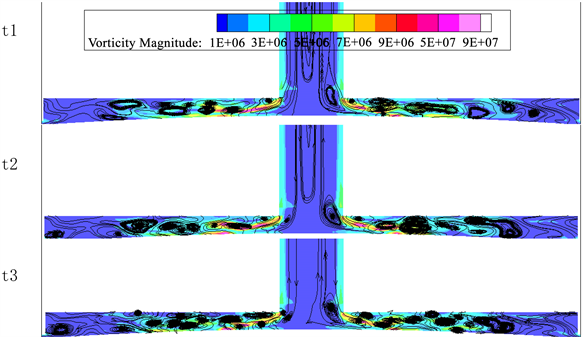Figure 3. Eccentricity (ε = 0.3) position distribution at 90˚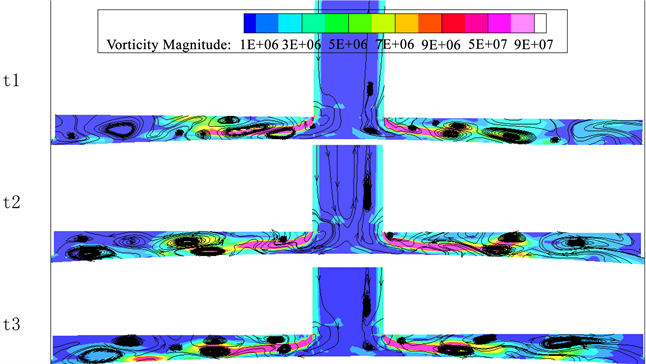Figure 4. Eccentricity (ε = 0.3) position distribution at 306˚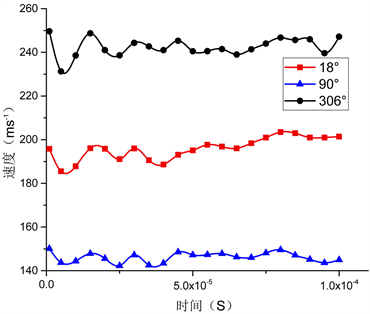(a)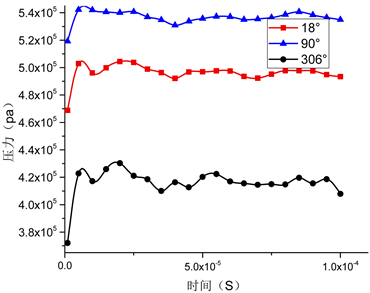(b)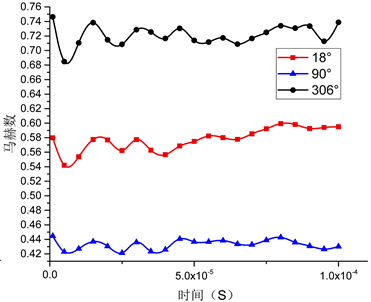(c)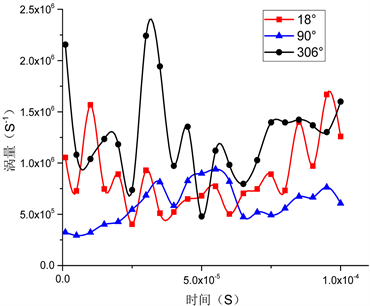(d)

Figure 5. The change of pressure, velocity and Maher number with time at the monitoring point at different throttle points at point-1

5. 结论(a)(b)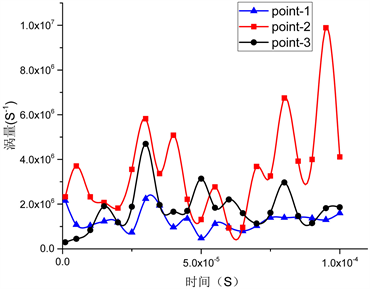(c)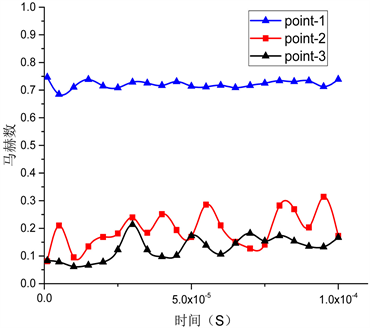(d)

Figure 6. The change of pressure, velocity, vorticity and Maher number at point 3 point at 306˚

1) 旋涡在空气静压轴承的节流腔中不断产生和脱落，在气膜间隙较大的位置漩涡强度较大，数量较小，在气膜厚度较小的位置，涡的数量较多，但强度较小。

2) 涡的产生和脱落都伴随着压力的波动，而速度的与压力的变化存在近似反比的关系。气膜厚度越大马赫数越大。

3) 越靠近节流孔的位置速度越大，马赫数也越大。

Dynamic Analysis of Radial Throttle Pinhole Hydrostatic Bearing Based on CFD[J]. 流体动力学, 2018, 06(04): 150-157. https://doi.org/10.12677/IJFD.2018.64019

1. 1. 冯慧成, 侯予, 陈汝刚, 等. 国内静压气体润滑技术研究进展[J]. 润滑与密封, 2011, 36(4): 108-113, 107.

2. 2. 贾晨辉, 杨伟, 邱明. 气体轴承的研究与发展[C]//中国轴承工业协会. 第七届中国轴承论坛论文集, 洛阳, 2014.

3. 3. 于贺春, 李欢欢, 胡居伟, 等. 单狭缝节流径向静压气体轴承的静态特性研究[J]. 液压与气动, 2015(6): 19-23.

4. 4. 黄首峰, 郭红, 张绍林, 等. 基于FLUENT的径向滑动轴承油膜压力仿真[J]. 机械设计与制造, 2012(10): 248-250.

5. 5. 胡俊宏, 迟青卓, 孙振鲁, 等. 基于FLUENT仿真的静压气浮轴承的研究[J]. 机床与液压, 2015, 43(4): 78-80.

6. 6. 孙雅洲, 卢泽生, 饶河清. 基于FLUENT软件的多孔质静压轴承静态特性的仿真与实验研究[J]. 机床与液压, 2007, 35(3): 170-172.

7. 7. 饶河清. 基于FLUENT软件的多孔质静压轴承的仿真与实验研究[D]: [硕士学位论文]. 哈尔滨: 哈尔滨工业大学, 2006.

8. 8. Renn, J.C. and Hsiao, C.H. (2004) Experimental and CFD Study on the Mass Flow-Rate Characteristic of Gas through Orifice-Type Restrictor in Aerostatic Bearings. Tribology International, 37, 309-315. https://doi.org/10.1016/j.triboint.2003.10.003

9. 9. Eleshaky, M.E. (2009) CFD Investigation of Pressure Depressions in Aero-static Circular Thrust Bearings. Tribology International, 42, 1108-1117. https://doi.org/10.1016/j.triboint.2009.03.011

10. 10. Gao, S.Y., et al. (2015) CFD Based Investigation on Influence of Orifice Chamber Shapes for the Design of Aerostatic Thrust Bearings at Ultra-High Speed Spindles. Tribology International, 92, 211-221. https://doi.org/10.1016/j.triboint.2015.06.020

11. 11. 李运堂, 蔺应晓, 朱红霞, 孙在. 基于大涡模拟静压气体推力轴承微幅自激振动特性分析[J]. 机械工程学报, 2013, 49(13): 56-62.